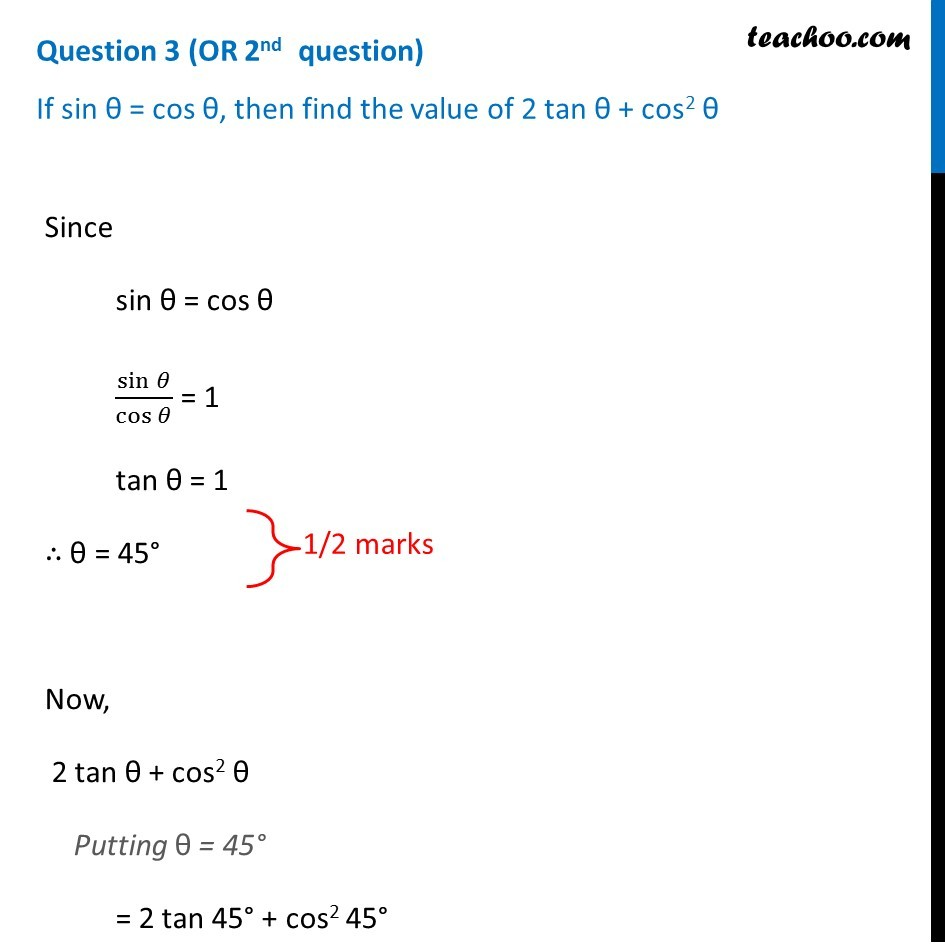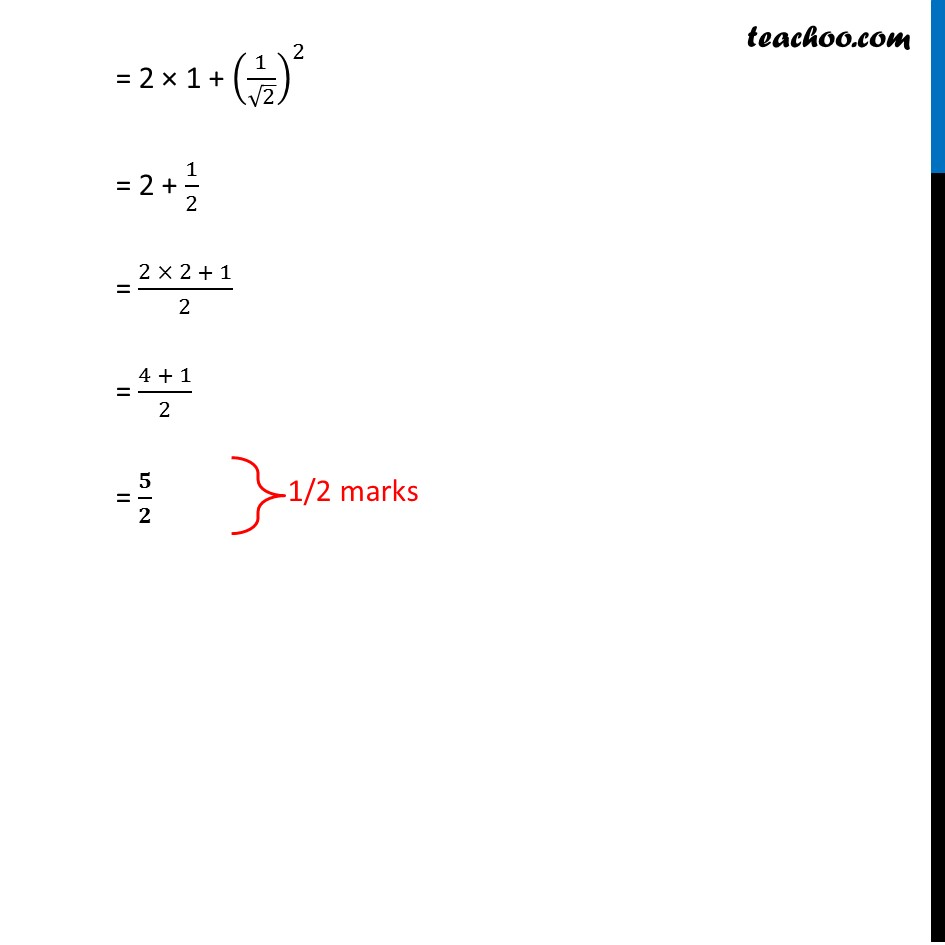Question 3 (OR 2 nd   question)

If sin θ = cos θ, then find the value of 2 tan θ + cos 2 θ1. Class 10
2. Solutions of Sample Papers for Class 10 Boards
3. CBSE Class 10 Sample Paper for 2019 Boards

Transcript

Question 3 (OR 2nd question) If sin θ = cos θ, then find the value of 2 tan θ + cos2 θ Since sin θ = cos θ sin⁡𝜃/cos⁡𝜃 = 1 tan θ = 1 ∴ θ = 45° Now, 2 tan θ + cos2 θ Putting θ = 45° = 2 tan 45° + cos2 45° = 2 × 1 + (1/√2)^2 = 2 + 1/2 = (2 × 2 + 1)/2 = (4 + 1)/2 = 𝟓/𝟐

CBSE Class 10 Sample Paper for 2019 Boards

Class 10
Solutions of Sample Papers for Class 10 Boards